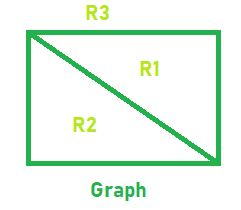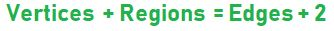# Program to find the number of region in Planar Graph

Given two integers V and E which represent the number of Vertices and Edges of a Planar Graph. The Task is to find the number of regions of that planar graph.

Planar Graph: A planar graph is one in which no edges cross each other or a graph that can be drawn on a plane without edges crossing is called planar graph.

Region: When a planar graph is drawn without edges crossing, the edges and vertices of the graph divide the plane into regions..

Examples:

Input: V = 4, E = 5
Output: R = 3Input: V = 3, E = 3
Output: R = 2

## Recommended: Please try your approach on {IDE} first, before moving on to the solution.

Approach: Euler found out the number of regions in a planar graph as a function of the number of vertices and number of edges in the graph i.e.Below is the implementation of the above approach:

## C++

 `// C++ implementation of the approach ` `#include ` `using` `namespace` `std; ` ` `  `// Function to return the number ` `// of regions in a Planar Graph ` `int` `Regions(``int` `Vertices, ``int` `Edges) ` `{ ` `    ``int` `R = Edges + 2 - Vertices; ` ` `  `    ``return` `R; ` `} ` ` `  `// Driver code ` `int` `main() ` `{ ` `    ``int` `V = 5, E = 7; ` ` `  `    ``cout << Regions(V, E); ` ` `  `    ``return` `0; ` `} `

## Java

 `// Java implementation of the approach ` `import` `java.io.*; ` ` `  `class` `GFG { ` ` `  `    ``// Function to return the number ` `    ``// of regions in a Planar Graph ` `    ``static` `int` `Regions(``int` `Vertices, ``int` `Edges) ` `    ``{ ` `        ``int` `R = Edges + ``2` `- Vertices; ` ` `  `        ``return` `R; ` `    ``} ` ` `  `    ``// Driver code ` `    ``public` `static` `void` `main(String[] args) ` `    ``{ ` ` `  `        ``int` `V = ``5``, E = ``7``; ` `        ``System.out.println(Regions(V, E)); ` `    ``} ` `} ` ` `  `// This code is contributed by akt_mit `

## Python3

 `# Python3 implementation of the approach  ` ` `  `# Function to return the number  ` `# of regions in a Planar Graph  ` `def` `Regions(Vertices, Edges) :  ` ` `  `    ``R ``=` `Edges ``+` `2` `-` `Vertices;  ` ` `  `    ``return` `R;  ` ` `  `# Driver code  ` `if` `__name__ ``=``=` `"__main__"` `:  ` ` `  `    ``V ``=` `5``; E ``=` `7``;  ` ` `  `    ``print``(Regions(V, E));  ` ` `  `# This code is contributed  ` `# by AnkitRai01 `

## C#

 `// C# implementation of the approach ` `using` `System; ` ` `  `class` `GFG { ` ` `  `    ``// Function to return the number ` `    ``// of regions in a Planar Graph ` `    ``static` `int` `Regions(``int` `Vertices, ``int` `Edges) ` `    ``{ ` `        ``int` `R = Edges + 2 - Vertices; ` ` `  `        ``return` `R; ` `    ``} ` ` `  `    ``// Driver code ` `    ``static` `public` `void` `Main() ` `    ``{ ` ` `  `        ``int` `V = 5, E = 7; ` `        ``Console.WriteLine(Regions(V, E)); ` `    ``} ` `} ` ` `  `// This code is contributed by ajit `

## PHP

 ` `

Output:

```4
```

Attention reader! Don’t stop learning now. Get hold of all the important DSA concepts with the DSA Self Paced Course at a student-friendly price and become industry ready.

My Personal Notes arrow_drop_upCheck out this Author's contributed articles.

If you like GeeksforGeeks and would like to contribute, you can also write an article using contribute.geeksforgeeks.org or mail your article to contribute@geeksforgeeks.org. See your article appearing on the GeeksforGeeks main page and help other Geeks.

Please Improve this article if you find anything incorrect by clicking on the "Improve Article" button below.

Improved By : AnkitRai01, jit_t, Code_Mech